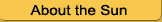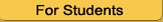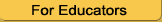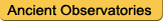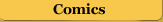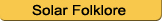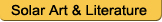Calculating the Sun's Angular Velocity

```
```

## What is Angular Velocity?

Angular velocity is how fast something travels in a circle (or on a sphere): it is the angle by which an object spins in a certain time -- its rotation rate.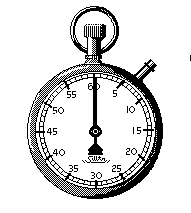Imagine a clock (the old-fashioned kind with hands). The minute hand goes around the clock, a circle -- 360 degrees, in 60 minutes. To find its angular velocity, or rotation rate, you need to divide the number of degrees by the number of minutes:

360 degrees / 60 minutes = 6 degrees per minute

So, the minute hand's angular velocity, or rate of rotation, is 6 degrees per minute.

```

```

```
```

## How Can I Determine the Sun's Angular Velocity?

We can use the movement of a sunspot to determine the Sun's rate of rotation, just like we used the minute hand of a clock. To do this accurately, you will have to use a transparent template for your sketches of the Sun's disk. The template has marked on it the degrees of longitude on a sphere.From your sketches, pick the sunspot group which is the most long-lived (that is, which appears in most of your sketches). Using your template, figure out how many degrees of Sun the spot group traveled across, and how long it took to move that far.

Sun's Angular velocity = degrees the spot has covered / time it took for the spot to travel

Sun's rotation rate = 360 degrees / angular velocity

## Going Further

If the Sun has been particularly active and you have sunspots sketched at different latitutes on the Sun's surface, compute the angular velocity for different sunspot groups which appear at varying latitudes. Do you get the same solar rotation rate for each set? If not, why not?```